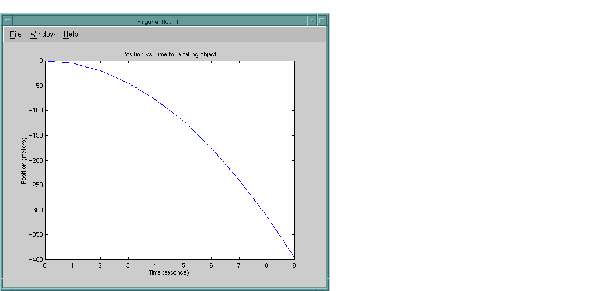External InterfacesExamples of Calling Engine Functions

This section contains examples that illustrate how to call engine functions from C and Fortran programs. The examples cover the following topics:

It is important to understand the sequence of steps you must follow when using the engine functions. For example, before using `engPutVariable`, you must create the matrix and populate it.

After reviewing these examples, follow the instructions in Compiling and Linking Engine Programs to build the application and test it. By building and running the application, you will ensure that your system is properly configured for engine applications.

Calling MATLAB from a C Application

This program, `engdemo.c`, illustrates how to call the engine functions from a stand-alone C program. For the Windows version of this program, see `engwindemo.c` in the `<matlab>\extern\examples\eng_mat` directory. Engine examples, like the MAT-file examples, are located in the `eng_mat` directory.

• ```/*
*  engdemo.c
*
*  This is a simple program that illustrates how to call the
*  MATLAB engine functions from a C program.
*
* Copyright (c) 1984-2000 The MathWorks, Inc.
* Revision: 1.8 \$
*/
#include <stdlib.h>
#include <stdio.h>
#include <string.h>
#include "engine.h"
#define  BUFSIZE 256

int main()

{
Engine *ep;
mxArray *T = NULL, *result = NULL;
char buffer[BUFSIZE];
double time = {0.0, 1.0, 2.0, 3.0, 4.0, 5.0, 6.0, 7.0,
8.0, 9.0};
/*
* Start the MATLAB engine locally by executing the string
* "matlab".
*
* To start the session on a remote host, use the name of
* the host as the string rather than \0.
*
* For more complicated cases, use any string with whitespace,
* and that string will be executed literally to start MATLAB.
*/
if (!(ep = engOpen("\0"))) {
fprintf(stderr, "\nCan't start MATLAB engine\n");
return EXIT_FAILURE;
}

/*
* PART I
*
* For the first half of this demonstration, we will send data
* to MATLAB, analyze the data, and plot the result.
*/

/*
* Create a variable for our data.
*/
T = mxCreateDoubleMatrix(1, 10, mxREAL);
memcpy((void *)mxGetPr(T), (void *)time, sizeof(time));

/*
* Place the variable T into the MATLAB workspace.
*/
engPutVariable(ep, "T", T);

/*
* Evaluate a function of time, distance = (1/2)g.*t.^2
* (g is the acceleration due to gravity).
*/
engEvalString(ep, "D = .5.*(-9.8).*T.^2;");

/*
* Plot the result.
*/
engEvalString(ep, "plot(T,D);");
engEvalString(ep, "title('Position vs. Time for a falling
object');");
engEvalString(ep, "xlabel('Time (seconds)');");
engEvalString(ep, "ylabel('Position (meters)');");

/*
* Use fgetc() to make sure that we pause long enough to be
* able to see the plot.
*/
printf("Hit return to continue\n\n");
fgetc(stdin);

/*
* We're done for Part I! Free memory, close MATLAB engine.
*/
printf("Done for Part I.\n");
mxDestroyArray(T);
engEvalString(ep, "close;");

/*
* PART II
*
* For the second half of this demonstration, we will request
* a MATLAB string, which should define a variable X.  MATLAB
* will evaluate the string and create the variable.  We
* will then recover the variable, and determine its type.
*/

/*
* Use engOutputBuffer to capture MATLAB output, so we can
* echo it back.
*/

engOutputBuffer(ep, buffer, BUFSIZE);
while (result == NULL) {
char str[BUFSIZE];

/*
* Get a string input from the user.
*/
printf("Enter a MATLAB command to evaluate.  This command
should\n");
printf("create a variable X.  This program will then
determine\n");
printf("what kind of variable you created.\n");
printf("For example: X = 1:5\n");
printf(">> ");

fgets(str, BUFSIZE-1, stdin);

/*
* Evaluate input with engEvalString.
*/
engEvalString(ep, str);

/*
* Echo the output from the command.  First two characters
* are always the double prompt (>>).
*/
printf("%s", buffer+2);

/*
* Get result of computation.
*/
printf("\nRetrieving X...\n");
if ((result = engGetVariable(ep,"X")) == NULL)
printf("Oops! You didn't create a variable X.\n\n");
else {
printf("X is class %s\t\n", mxGetClassName(result));
}
}

/*
* We're done! Free memory, close MATLAB engine and exit.
*/
printf("Done!\n");
mxDestroyArray(result);
engClose(ep);

return EXIT_SUCCESS;
}
```

The first part of this program launches MATLAB and sends it data. MATLAB then analyzes the data and plots the results.The program then continues with

• ```Press Return to continue
```

Pressing Return continues the program.

• ```Done for Part I.
Enter a MATLAB command to evaluate.  This command should
create a variable X.  This program will then determine
what kind of variable you created.
For example: X = 1:5
```

Entering `X = 17.5` continues the program execution.

• ```X = 17.5

X =

17.5000

Retrieving X...
X is class double
Done!
```

Finally, the program frees memory, closes the MATLAB engine, and exits.GUI-Intensive Applications Calling MATLAB from a Fortran Application© 1994-2005 The MathWorks, Inc.